# 1 卷积神经网络

### 1.1 用简单的话来解释一下 卷积神经网络：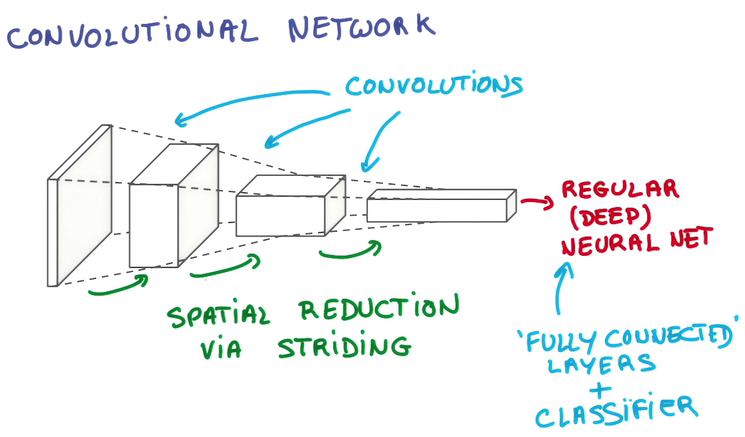## 1.2 假设人脑cnn识别美女# 1.3 CNN的重要组成

## 1.3.1 卷积核 (patch/keruel)

• stride patch每次跨多少像素去抽离信息
• Feature Map：每层Conv网络，因为它们将前一层的feature映射到后一层（Output map）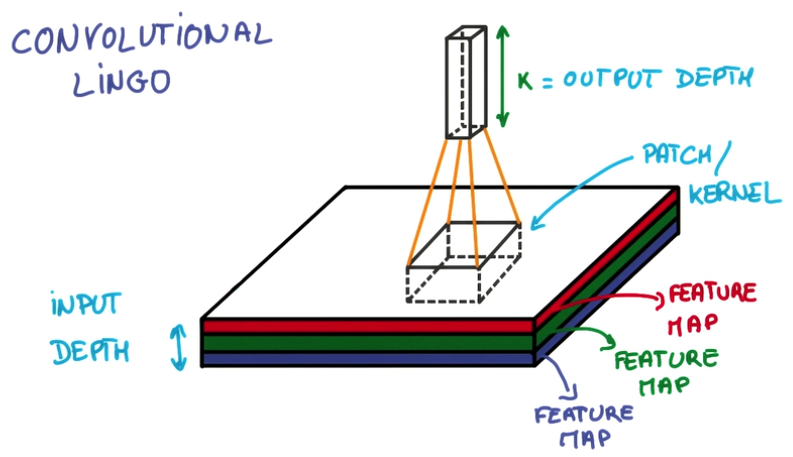patch 为卷积核，有长宽高，K为对抽取的信息进行像素的加权求和返回的信息，厚度变厚了。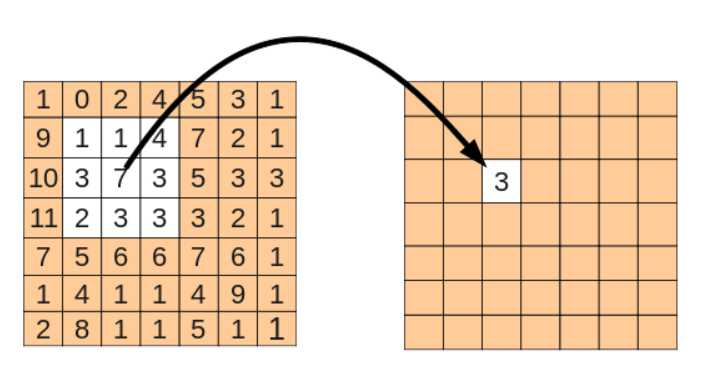## 1.3.2 池化（pooling）

pooling层也就是下采样也就是减小临近像素的差异值，并缩小或者不缩小图片（一般选择缩小）。pooling 分多种例如：

• Max Pooling 在一个卷积层的输出层上取一个切片，取其中最大值代表这个切片
• Average Pooling 在卷积层输出中，取切片，取平均值代表这个切片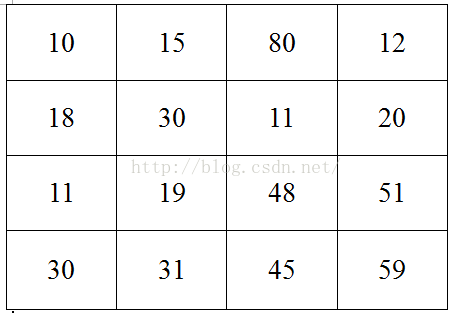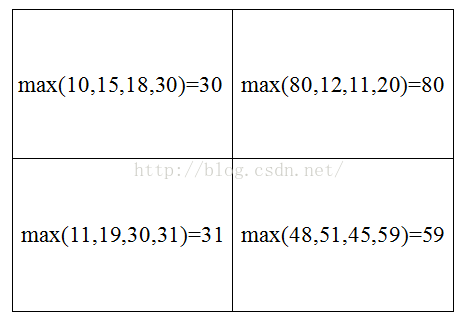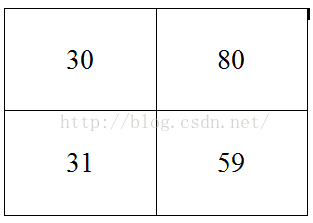## 2 卷积神经网络之MNIST

# -*- coding: utf-8 -*-
# 手写数字识别
# 准确度 0.9679
import 手写数字识别.input_data
import tensorflow as tf

def compute_accuracy(v_xs,v_ys):
# 定义 prediction 为全局变量
global prediction
# 将 xs data 在 prediction 中生成预测值，预测值也是一个 1*10 的矩阵 中每个值的概率，并不是一个0-9 的值，是0-9 每个值的概率 ，比如说3这个位置的概率最高，那么预测3就是这个图片的值
y_pre = sess.run(prediction, feed_dict={xs: v_xs,keep_prob: 1})
# 对比我的预测值y_pre 和真实数据 v_ys 的差别
correct_prediction = tf.equal(tf.argmax(y_pre,1), tf.argmax(v_ys,1))
# 计算我这一组数据中有多少个预测是对的，多少个是错的
accuracy = tf.reduce_mean(tf.cast(correct_prediction, tf.float32))
# result 是一个百分比，百分比越高，预测越准确
result = sess.run(accuracy, feed_dict={xs: v_xs, ys: v_ys,keep_prob: 1})
return result

#我们定义Weight变量，输入shape，返回变量的参数。其中我们使用tf.truncted_normal产生随机变量来进行初始化
def weight_variable(shape):
initial = tf.truncated_normal(shape,stddev=0.1)
return tf.Variable(initial)
#定义biase变量，输入shape ,返回变量的一些参数。其中我们使用tf.constant常量函数来进行初始化
def bias_variable(shape):
#定义成 0.1之后才会从0.1变到其他的值， bias通常用正直比较好，所以我们用0.1
initial = tf.constant(0.1,shape=shape)
return tf.Variable(initial)

#定义卷积，tf.nn.conv2d函数是tensoflow里面的二维的卷积函数，x是图片的所有参数，W是此卷积层的权重
def conv2d(x,W):

#定义池化pooling  x 为conv2d 的返回 ,在pooling 阶段图片的长和宽被减小
def max_poo_2x2(x):

xs = tf.placeholder(tf.float32,[None, 784]) #图像输入向量  每个图片有784 （28 ＊28） 个像素点
ys = tf.placeholder(tf.float32, [None,10]) #每个例子有10 个输出
keep_prob = tf.placeholder(tf.float32)

# 处理输入图片的信息 把xs的形状变成[-1,28,28,1]，-1代表先不考虑输入的图片例子多少这个维度，28 28 代表的是长和宽 后面的1是channel的数量，因为我们输入的图片是黑白的，因此channel是1，例如如果是RGB图像，那么channel就是3
xs_image = tf.reshape(xs,[-1,28,28,1])
# print(xs_image.shape)

# 第一层##
# 定义本层的Weight,本层我们的卷积核patch的大小是5x5，因为黑白图片channel是1 是图片的厚度 所以输入是1 彩色的厚度是3，输出是32个featuremap
W_conv1 = weight_variable([5,5,1,32])
# 大小是32个长度，因此我们传入它的shape为
b_conv1= bias_variable()
# 输出图片的大小没有变化依然是28x28，只是厚度变厚了，因此现在的输出大小就变成了28x28x32
h_conv1 =tf.nn.relu(conv2d(xs_image,W_conv1) + b_conv1)
# 经过pooling的处理，输出大小就变为了14x14x32
h_pool1 = max_poo_2x2(h_conv1)

# 第二层##
# 定义本层的Weight,本层我们的卷积核patch的大小是5x5，32 是图片的厚度，输出是64个featuremap
W_conv2 = weight_variable([5,5,32,64])
# 大小是64个长度，因此我们传入它的shape为
b_conv2= bias_variable()
# 输出图片的大小没有变化依然是14x14，只是厚度变厚了，因此现在的输出大小就变成了14x14x64
h_conv2 =tf.nn.relu(conv2d(h_pool1,W_conv2) + b_conv2)
# 经过pooling的处理，输出大小就变为了7x7x64
h_pool2 = max_poo_2x2(h_conv2)

#func1 layer##
# 参考上面注释
W_fc1 = weight_variable([7*7*64,1024])
b_fc1 = bias_variable()
# 通过tf.reshape()将h_pool2的输出值从一个三维的变为一维的数据, -1表示先不考虑输入图片例子维度, 将上一个输出结果展平，
h_pool2_flat = tf.reshape(h_pool2,[-1,7*7*64]) #[n_samples,7,7,64]->>[n_samples,7*7*64]

# 将展平后的h_pool1_flat与本层的W_fc1相乘（注意这个时候不是卷积了）
h_fc1 = tf.nn.relu(tf.matmul(h_pool2_flat,W_fc1)+b_fc1)
# 考虑过拟合问题，可以加一个dropout的处理
h_fc1_drop = tf.nn.dropout(h_fc1,keep_prob)

#func2 layer##
W_fc2 = weight_variable([1024,10])
b_fc2 = bias_variable()

# 预测值，prediction 用softmax 处理 计算概率
prediction = tf.nn.softmax(tf.matmul(h_fc1_drop,W_fc2)+b_fc2)

#loss函数（即最优化目标函数）选用交叉熵函数。交叉熵用来衡量预测值和真实值的相似程度，如果完全相同，它们的交叉熵等于零 ,所以loss 越小 学的好
#分类一般都是 softmax＋ cross_entropy
cross_entropy = tf.reduce_mean(-tf.reduce_sum(ys * tf.log(prediction),
reduction_indices=))
# cross_entropy = -tf.reduce_sum(ys*tf.log(prediction))

sess = tf.Session()

# 初始化变量
init= tf.global_variables_initializer()
sess.run(init)

for i in range(1000):
#开始train，每次只取100张图片，免得数据太多训练太慢
batch_xs, batch_ys = mnist.train.next_batch(100)
sess.run(train_step, feed_dict={xs: batch_xs, ys: batch_ys,keep_prob: 0.5})
if i % 50 == 0:
print(compute_accuracy(
mnist.test.images, mnist.test.labels))